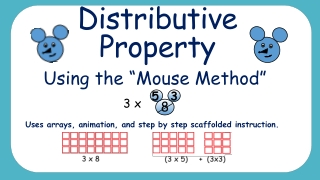Download PresentationDistributive Property

# Distributive Property

Download Presentation## Distributive Property

- - - - - - - - - - - - - - - - - - - - - - - - - - - E N D - - - - - - - - - - - - - - - - - - - - - - - - - - -
##### Presentation Transcript

1. Distributive Property 3 5 3 x Using the “Mouse Method” Uses arrays, animation, and step by step scaffolded instruction. 8

2. Distributive Property • The distributive property allows you to decompose (break down) one factor. Then you can use the smaller known facts to find products. 8 3

3. The Distributive Property We can use the Distributive Property of Multiplication to make division easier! Let’s look at it step by step! How many rows are in this array? How many columns are in this array? 3 8 8 3

4. The Distributive Property Great! So this array represents: 3 x 8 Now click to see how the mouse will split this array! 8 3

5. The Distributive Property

6. The Distributive Property The mouse split this array into two! What is the new array on the left? What is the new array on the right? 3 x 5 3x 3

7. The Distributive Property So we began with 3x8 The array was split into (3x5) and (3x3)

8. The Distributive Property 3x8 (3x5) and (3x3) 9 Multiply: 3x3 = 3x5 = 15 Add the products: 24 15 + 9 = so 3x8= 24 YOU DID IT!

9. The Distributive Property 6 Let’s look at a new array How many rows are in this array? How many columns are in this array? 9 9 6

10. The Distributive Property Great! So this array represents: 6 X 9 Now click to see how the mouse will split this array! 9 6

11. The Distributive Property The mouse split this array into two! What is the new array on the left? What is the new array on the right? 6 x 4 6x 5

12. The Distributive Property So we began with 6x9 The array was split into (6x4) and (6x5)

13. The Distributive Property 6x9 (6x4) and (6x5) 6x5 = 30 Multiply: 6x4 = 24 Add the products: 54 24 + 30 = so YOU DID IT! 6x9= 54

14. The Distributive Property Let’s be more efficient by using just the mouse! 4 x 9 = ?

15. The Distributive Property Let’s be more efficient by using just the mouse! 4 x = ? 9 1.Change one number with mouse ears!

16. The Distributive Property Let’s be more efficient by using just the mouse! 5 4 4 x = ? 9 2.The ears add up to the face number (split one factor)

17. The Distributive Property Let’s be more efficient by using just the mouse! 4 5 4 x = ? 3.Multiply the other factor by each ear number 9 (4 x4) + (4x5)

18. The Distributive Property 3.Multiply the other factor by each ear number 5 4 4 x = ? (4 x4) + (4x5) 4.Add the products together 9 36 16 + 20 = so 36 4 x 9 = YOU DID IT!

19. The Distributive Property 15 x 6 = ? 1.Change one number with mouse ears!

20. The Distributive Property 10 10 5 5 x 6 = ? x 6 = ? 15 15 2.Change one number with mouse ears! (Split one factor)

21. The Distributive Property x 6 = ? 10 5 3.Multiply the other factor by each ear number (10 x6) + (5x6) 4.Add the products together 15 90 60 + 30 = so 90 15 x 6= YOU DID IT!

22. The Distributive Property of Multiplication Multiplying two factors together gives the same result as breaking one factor into two addends, multiplying both addends with the remaining factor, then adding both products together.

23. The Distributive Property of Multiplication We use the Distributive Property to “break” one array into two smaller arrays to make multiplication easier! + = (3 x 5) + (3x3) 3 x 8